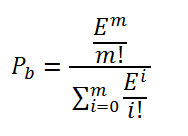# Erlang B Formula

Posted in Operations and Supply Chain Terms, Total Reads: 4485

## Definition: Erlang B Formula

The Erlang B formula that is also known as Erlang loss formula calculates the blocking probability of a service system generally telephone networks. For example, in case of a telephone network, the blocking probability is the probability of a call being lost due to all attending servers being busy and there is no buffer space for waiting or queuing the calls.

The formula is given as follows,where,

Pb = Probability of blocking

E = the total amount of traffic in Erlangs given by λh, where λ= rate of arrival of new calls & h= average call time

m = number of server or resources in the service system

and it is assumed that the calls received follow a Poisson distribution.

The Erlang B formula is mostly used to calculate the minimum number of servers of resources that would be required to achieve a required probability of service delivery during peak times.

Browse the definition and meaning of more terms similar to Erlang B Formula. The Management Dictionary covers over 7000 business concepts from 6 categories.

Search & Explore : Management Dictionary

Similar Definitions from same Category: